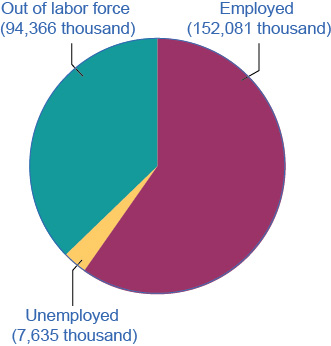# How Economists Define and Compute Unemployment Rate

## Calculating the Unemployment Rate

Figure shows the three-way division of the 16-and-over population. In January 2017, about 62.9% of the adult population was "in the labor force"; that is, people are either employed or without a job but looking for work. We can divide those in the labor force into the employed and the unemployed. Table shows those values. The unemployment rate is not the percentage of the total adult population without jobs, but rather the percentage of adults who are in the labor force but who do not have jobs:Employed, Unemployed, and Out of the Labor Force Distribution of Adult Population (age 16 and older), January 2017 The total adult, working-age population in January 2017 was 254.1 million. Out of this total population, 152.1 were classified as employed, and 7.6 million were classified as unemployed. The remaining 94.4 were classified as out of the labor force. As you will learn, however, this seemingly simple chart does not tell the whole story.
 Total adult population over the age of 16 254.082 million In the labor force 159.716 million (62.9%) Employed 152.081 million Unemployed 7.635 million Out of the labor force 94.366 million (37.1%)

In this example, we can calculate the unemployment rate as 7.635 million unemployed people divided by 159.716 million people in the labor force, which works out to a 4.8% rate of unemployment. The following Work It Out feature will walk you through the steps of this calculation.

## Calculating Labor Force Percentages

How do economists arrive at the percentages in and out of the labor force and the unemployment rate? We will use the values in Table to illustrate the steps.

To determine the percentage in the labor force:

Step 1. Divide the number of people in the labor force (159.716 million) by the total adult (working-age) population (254.082 million).

Step 2. Multiply by 100 to obtain the percentage.

To determine the percentage out of the labor force:

Step 1. Divide the number of people out the labor force (94.366 million) by the total adult (working-age) population (254.082 million).

Step 2. Multiply by 100 to obtain the percentage.

To determine the unemployment rate:

Step 1. Divide the number of unemployed people (7.635 million) by the total labor force (157 million).

Step 2. Multiply by 100 to obtain the rate.